# Decimals Class 6 Maths Notes - Chapter 8

The numbers used to represent numbers smaller than unit 1 are called decimal numbers. The decimal point or the period plays a significant part in a Decimal Number. This period separates the fractional part and whole number part in a decimal number. Place value of a digit can be defined as the value of a digit as per the place of that digit in a number.

## Decimals Examples

Let us consider a decimal number 0.5694 to see the different place values of each digit:

• The value of the digit at the first place after the decimal point is tenths place value. Tenths can be calculated as 1 unit divided in 10 equal parts i.e 0.1. Therefore, considering this there are 5 tenths in the number 0.5694.
• The value of the digit at the second place after the decimal point is hundredths place value. The number in that place tells you how many hundredths are there. Hundredths can be calculated as 1 unit divided in100 equal parts i.e 0.01. Therefore, considering this there are 6 hundredths in the number 0.5694
• The value of the digit at the third place after the decimal point is thousandths place value. The number in that place tells you how many thousandths are present. Thousandths can be calculated as 1 unit divided in1000 equal parts i.e 0.001. Therefore, considering this there are 9 thousandths in the number 0.5694
• Ten-thousandths
• The value of the digit at the fourth place after the decimal point is ten-thousandths place value. The number in that place tells you how many ten-thousandths are present. Ten-thousandths can be calculated as 1 unit divided into 10000 equal parts i.e 0.0001. Therefore, considering this there are 4 thousandths in the number 0.5694

### Introduction to Decimals

• Decimals are used in many ways in our lives.
• For example, in representing units of money, length and weight.
• Example: Price of the book is Rs. 34.5

To know more about Decimals, visit here.

### Representing Decimals on a Number Line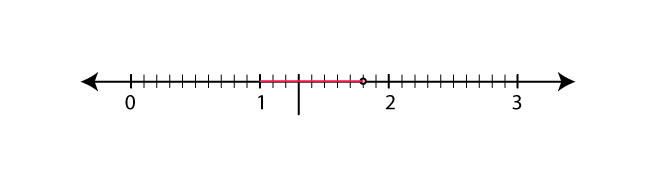Example: Represent 1.3 on the number line.

• 1.3 is more than 1 but less than 2.
• Divide each unit length into 10 equal parts. From 1, take 3 parts to the right and we will get 1.3.

To know more about Representation of Decimals on Number Line, visit here.

#### For more information on Fractions and Decimals, watch the below video### Inter-Conversion of Fractions and Decimals

Fractions as decimals

• 12/5 = 24/10 = (20+4) / 10 = 20/10 + 4/10 = 2+0.4 = 2.4

Decimals as fractions

• 21.2 = 21 + (2/10) = (210/10) + (2/10) = (212/10) = (106/5)

To know more about Conversion of Decimal to Fractions, visit here.

### Recurring and Terminating Decimals

• A terminating decimal is a decimal number which has finite number of digits after the decimal.
Example: 0.35
• A recurring decimal is one where its decimal representation becomes periodic or same sequence of digits keeps repeating indefinitely.
Example: 31.213333…

## Decimal 1 vs. Decimal 2

### Comparing Decimals

• Any two decimal numbers can be compared by comparing their whole part and decimal parts.
• If the whole parts are equal then the tenth parts can be compared and so on.
• Comparison when whole parts equal
Compare 22.3 and 22.5
Whole parts are equal. Hence we compare tenths part.
22.3=22 + 3/10
22.5 = 22 + 5/10
3/10<5/10
∴ 22.3 < 22.5.
• Comparison whole parts are unequal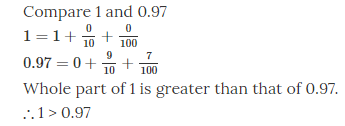To know more about Comparing Decimal Numbers, visit here.

## Let’s Give Names to Numbers

### Tenths and Hundredths

• 191.8 191 + (8/10)
⇒ 8 occupies the tenths position.
• Move the tenths place to the right by one unit, the place value decreases further to 1/100th (hundredth) place.
• 191.86 191 + 8/10 + 6/100
⇒ 6 occupies the hundredths place

### Place values

• Place value gives a value of the number depending on its location.
• Place value table of 5.2 is as shown below.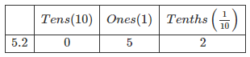## Many Many Many Points Together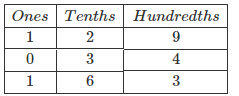Thus, 1.29 + 0.34 = 1.63

### Subtraction of Decimals

Subtraction of 5.25 from 8.28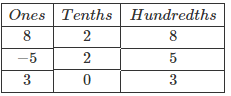Thus, 8.28 – 5.25 = 3.03

### Multiplication of Decimals

Multiplication of 2.8 and 7

• 2.8 × 7 (There is only one digit to the right of the decimal point in 2.8)
• 28 × 7 (Ignoring the decimals)
• 28 × 7 = 196
• Now bring the decimal back after one digit from left and thus answer is 19.6

### Division of Decimals

Divide 3.4 by 2

• 3.4/2 = Quotient
• Ignore the decimal and divide the numerator by the denominator. Here, quotient = 34/2 = 17
• Since there is only one digit to the right of the decimal, put the decimal after one digit from left in the quotient. Therefore, quotient becomes 1.7

To know more about Multiplication and Division of Decimals, visit here.

## Points on How to Use the Points

### Point Shift Trick

Division: Point is shifted to the left by the number of zeroes in the denominator.

• 150/100=1.50 ( Shifting decimal by 2 points to the left )
• 1.5/1000=0.0015 ( Shifting decimal by 3 points to the left )

### Decimals in Length Measurement

• 1m = 100cm
• 1cm = 1/100m = 0.01m ( Move decimal to left by 2 units in the numerator as there are two zeroes in the denominator )
• 150cm = 150/100m = 1.5m

### Decimals in Weight Measurement

• 1 kg = 1000 g
• 1 g = 1/1000 kg
• 298 g = 298/1000 kg = 0.298 kg ( Move the decimal to the left by 3 places in the numerator as there are 3 zeroes in the denominator )

 Decimal Fractions Fractions

## Frequently Asked Questions on CBSE Class 6 Maths Notes Chapter 8 Decimals

Q1

### What are termination decimals?

A terminating decimal is one which can be expressed in a finite number of figures or for which all figures to the right of some place are zero: compare repeating decimal.

Q2

### What are the importance of fractions?

1. Determining the part of a number 2. Probability 3. Ratio calculations 4. Algebraic equations 5. Percentage determination

Q3

### What are the uses of decimals?

Decimal numbers are used in situations where more precision is required than the whole numbers can provide